# Determining x and y Intercepts From a Graph Worksheet

For problems 1 through 10, find the y intercept of the line. For problems 11 through 20, find the x intercept of the line.

Click “Show Answer” underneath the problem to see the answer. Or click the “Show Answers” button at the bottom of the page to see all the answers at once.

## Example:

`Equation: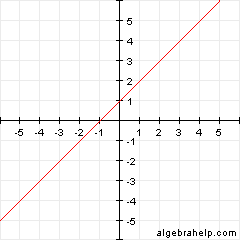`
`Answer: 1`

## Quiz:

1. `Equation: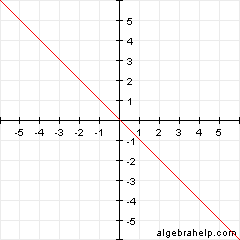`
`Answer: 0`
2. `Equation: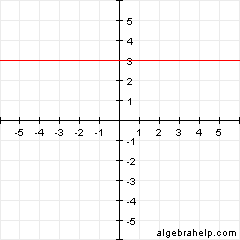`
`Answer: 3`
3. `Equation: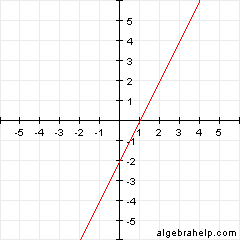`
`Answer: -2`
4. `Equation: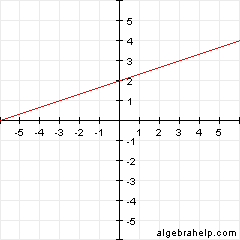`
`Answer: 2`
5. `Equation: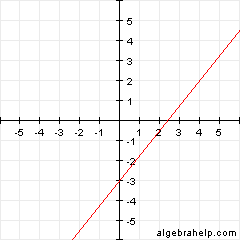`
`Answer: -3`
6. `Equation: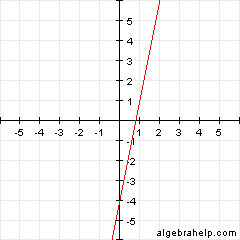`
`Answer: -4`
7. `Equation: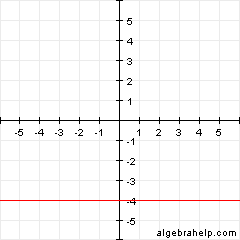`
`Answer: -4`
8. `Equation: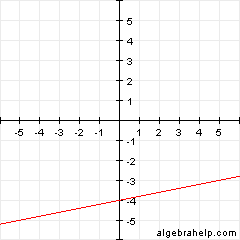`
`Answer: -4`
9. `Equation: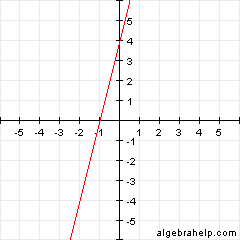`
`Answer: 4`
10. `Equation: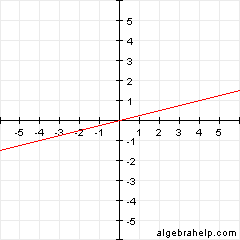`
`Answer: 0`
11. `Equation: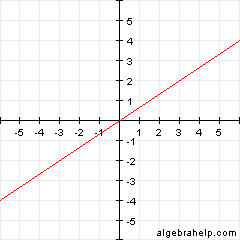`
`Answer: 0`
12. `Equation: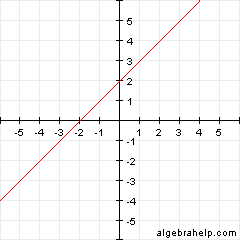`
`Answer: -2`
13. `Equation: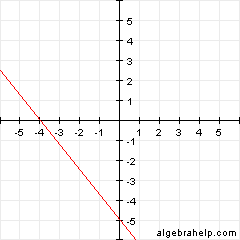`
`Answer: -4`
14. `Equation: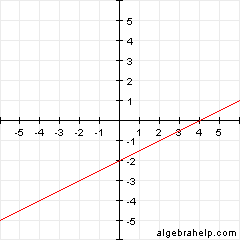`
`Answer: 4`
15. `Equation: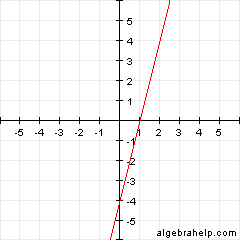`
`Answer: 1`
16. `Equation: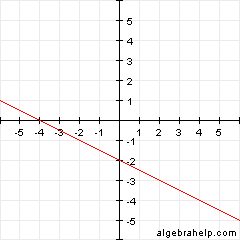`
`Answer: -4`
17. `Equation: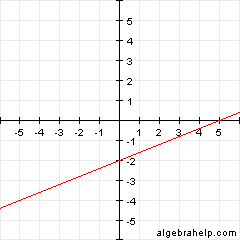`
`Answer: 5`
18. `Equation: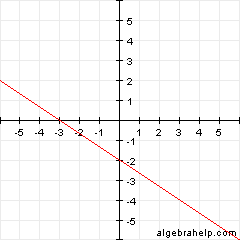`
`Answer: -3`
19. `Equation: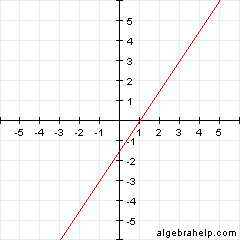`
`Answer: 1`
20. `Equation: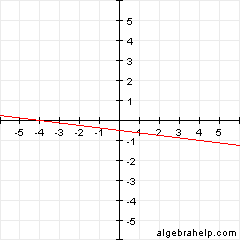`
`Answer: -4`

## Related Pages

PrintFreeGraphPaper.com provides printable PDFs of various types of graph paper.

## Tutoring

Looking for someone to help you with algebra? At Wyzant, connect with algebra tutors and math tutors nearby. Prefer to meet online? Find online algebra tutors or online math tutors in a couple of clicks.

Scroll to Top